# can anyone please help me with this question? I know it is tedious and hard but...

can anyone please help me with this question? I know it is tedious and hard but if you can help me with full step by step solutions, I will really be grateful. I'm also sorry that this question has multiple parts.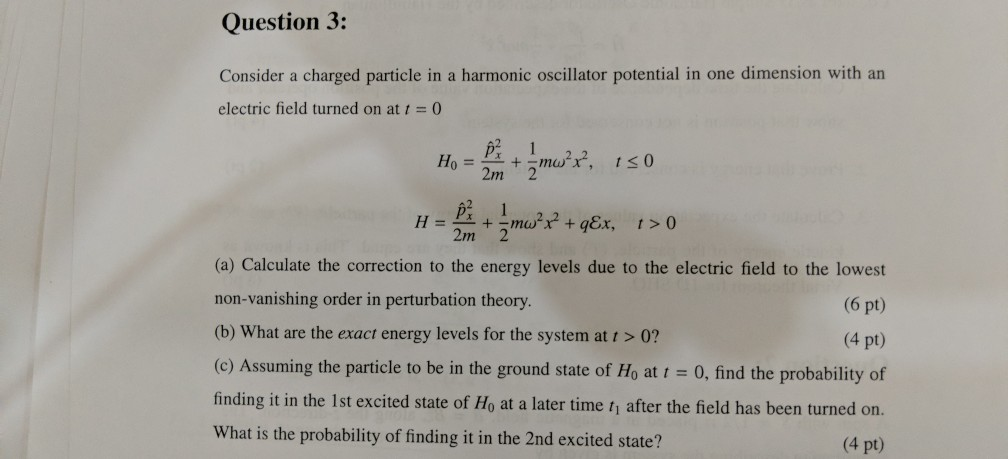Question 3: Consider a charged particle in a harmonic oscillator potential in one dimension with an electric field turned on at t0 2m 2 10 2m 2 (a) Calculate the correction to the energy levels due to the electric field to the lowest non-vanishing order in perturbation theory (b) What are the exact energy levels for the system at t > 0? (c) Assuming the particle to be in the ground state of Ho at t-0, find the probability of finding it in the 1st excited state of Ho at a later time tj after the field has been turned on. What is the probability of finding it in the 2nd excited state? (6 pt) (4 pt) (4 pt)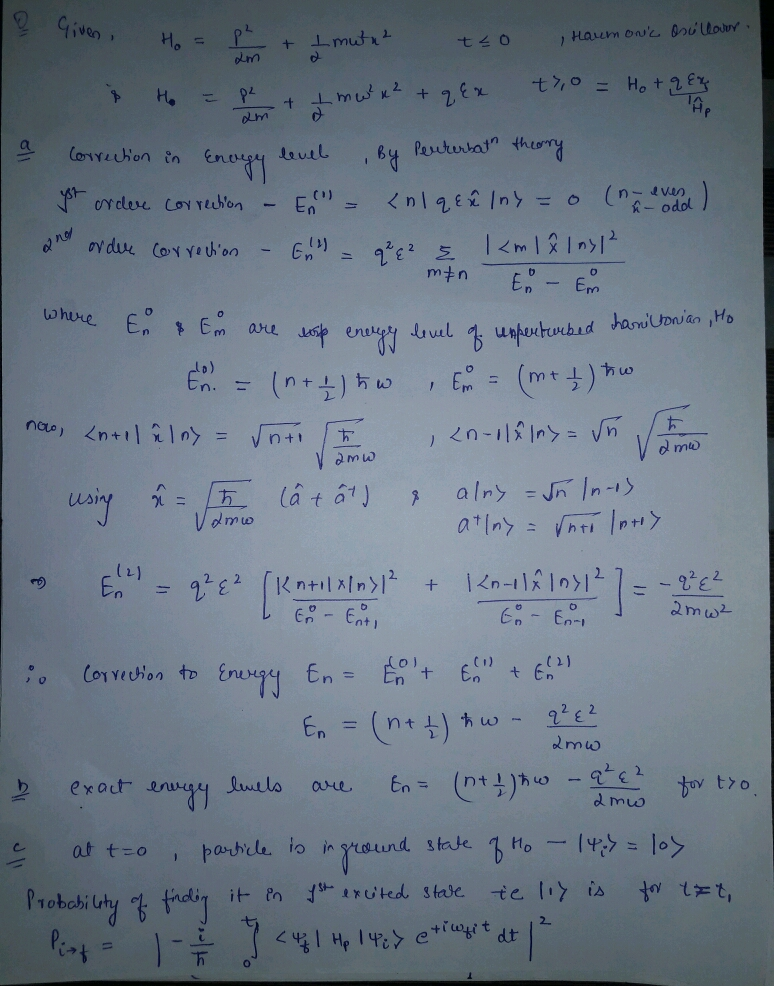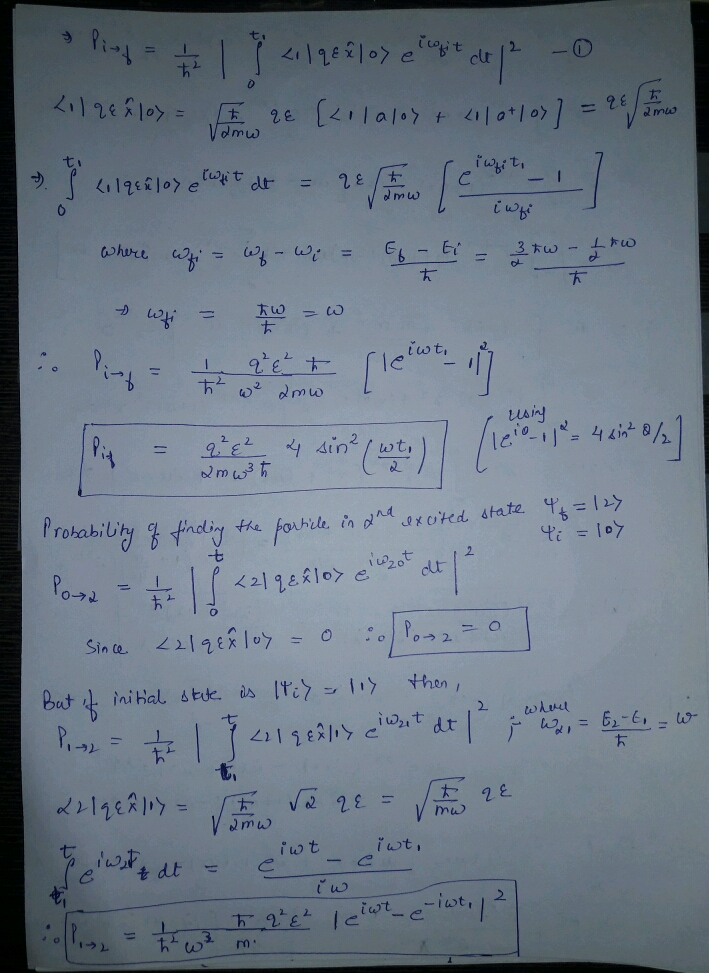#### Earn Coin

Coins can be redeemed for fabulous gifts.

Similar Homework Help Questions
• ### please solve with explanations 3. (20 pts) A particle of mass m and charge q is in a one dimensional harmonic oscillato...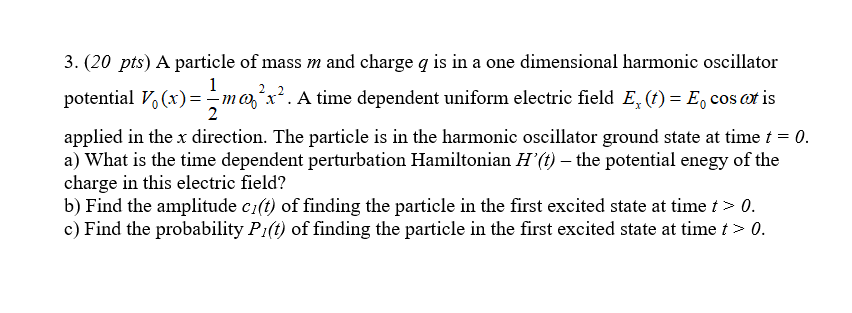please solve with explanations 3. (20 pts) A particle of mass m and charge q is in a one dimensional harmonic oscillator potential ()1ma'. A time dependent uniform electric field E, ()E, os eris 2 applied in the x direction. The particle is in the harmonic oscillator ground state at time a) What is the time dependent perturbation Hamiltonian H'(t) - the potential enegy of the charge in this electric field? b) Find the amplitude ci(t) of finding the particle...

• ### A particle of charge q and mass m is bound in the ground state of a one-dimensional harmonic osci...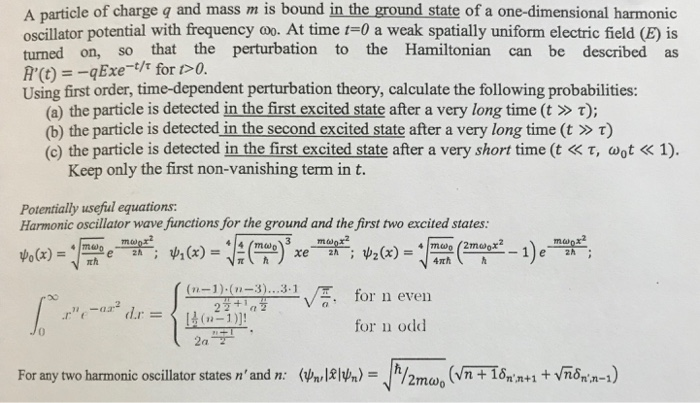A particle of charge q and mass m is bound in the ground state of a one-dimensional harmonic oscillator potential with frequency oo. At time t-0 a weak spatially uniform electric field (E) is turned on, so that the perturbation to the Hamiltonian can be described as R'(t) =-q Exe-t/t for t> 0. Using first order, time-dependent perturbation theory, calculate the following probabilities: (a) the particle is detected in the first excited state after a very long time (t »...

• ### 1. Consider a spin-0 particle of mass m and charge q moving in a symmetric three-dimensional harm...1. Consider a spin-0 particle of mass m and charge q moving in a symmetric three-dimensional harmonic oscillator potential with natural frequency W.Att-0 an external magnetic field is turned on which is uniform in space but oscillates with temporal frequency W as follows. E(t)-Bo sin(at) At time t>0, the perturbation is turned off. Assuming that the system starts off at t-0 in the ground state, apply time-dependent perturbation theory to estimate the probability that the system ends up in an...

• ### Problem 2. (30 points) (a) (3 points) The Stark effect (shift of energy levels by a constant external electric field) i...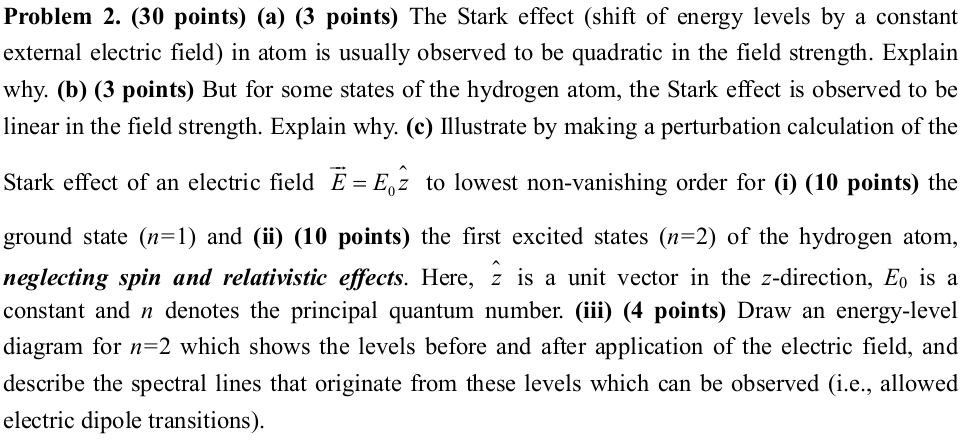Problem 2. (30 points) (a) (3 points) The Stark effect (shift of energy levels by a constant external electric field) in atom is usually observed to be quadratic in the field strength. Explain why. (b) (3 points) But for some states of the hydrogen atom, the Stark effect is observed to be linear in the field strength. Explain why. (c) Ilustrate by making a perturbation calculation of the Stark effect of an electric field E Ez to lowest non-vanishing order...

• ### 4. This problems deals with sudden approximation, please do not use perturbation theory. (a) (1 p...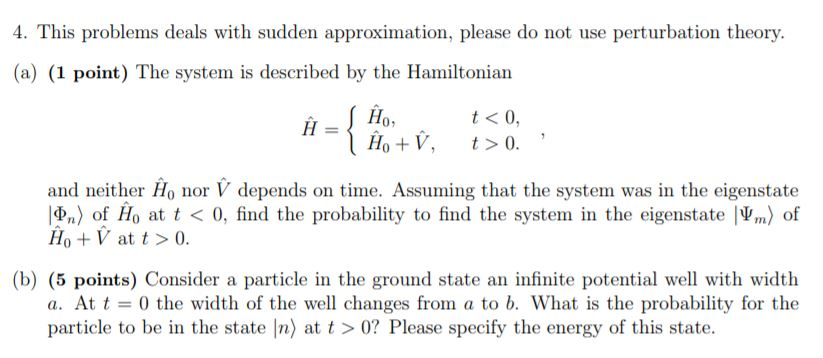4. This problems deals with sudden approximation, please do not use perturbation theory. (a) (1 point) The system is described by the Hamiltonian t < 0, Ho+V, t>0 - and neither Ho nor V depends on time. Assuming that the system was in the eigenstate n) of Ho at t < 0, find the probability to find the system in the eigenstate V) of Ho V at t>0. (b) (5 points) Consider a particle in the ground state an infinite...

• ### QUANTUM MECHANICS Problem 4 Consider a one-dimensional charged harmonic oscillator. Let the coordinate be, charge be...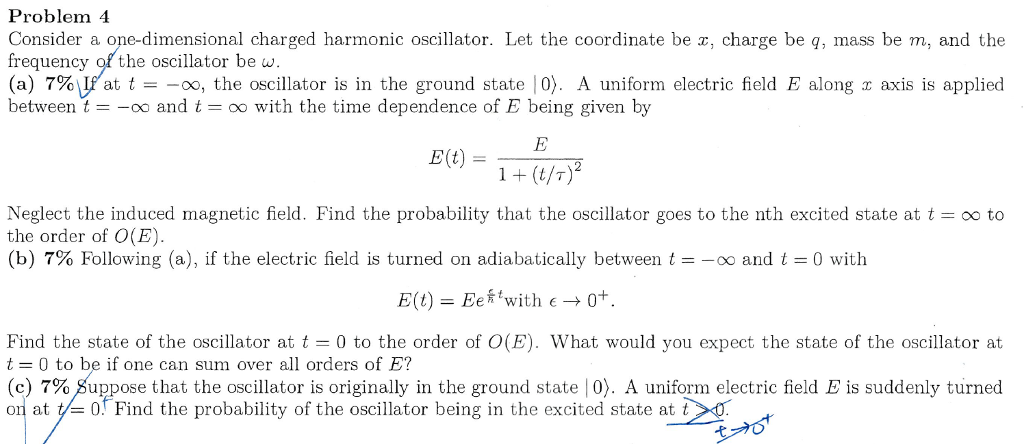QUANTUM MECHANICS Problem 4 Consider a one-dimensional charged harmonic oscillator. Let the coordinate be, charge be q, mass be m, and the frequency of the oscillator be u. (a) 79 rat t =-oo, the oscillator is in the ground state 10). A uniform electric field E along x axis is applied betweentoo andtoo with the time dependence of E being given by E(t) ー(t/ア Neglect the induced magnetic field. Find the probability that the oscillator goes to the nth excited...

• ### I am really struggling with quantum. Can someone please help me with those questions 4.2. Using...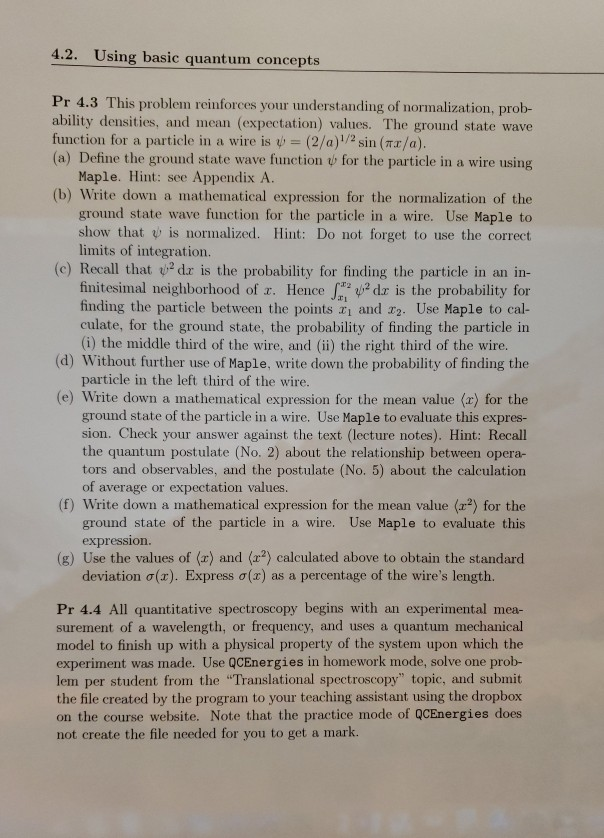I am really struggling with quantum. Can someone please help me with those questions 4.2. Using basic quantum concepts Pr 4.3 This problem reinforces your understanding of normalization, prob- ability densities, and mean (expectation) values. The ground state wave function for a particle in a wire is = (2/a)/2 sin(x/a). (a) Define the ground state wave function for the particle in a wire using Maple. Hint: see Appendix A. (b) Write down a mathematical expression for the normalization of the...

• ### qm 2019.3 3. The Hamiltonian corresponding to the magnetic interaction of a spin 1/2 particle with...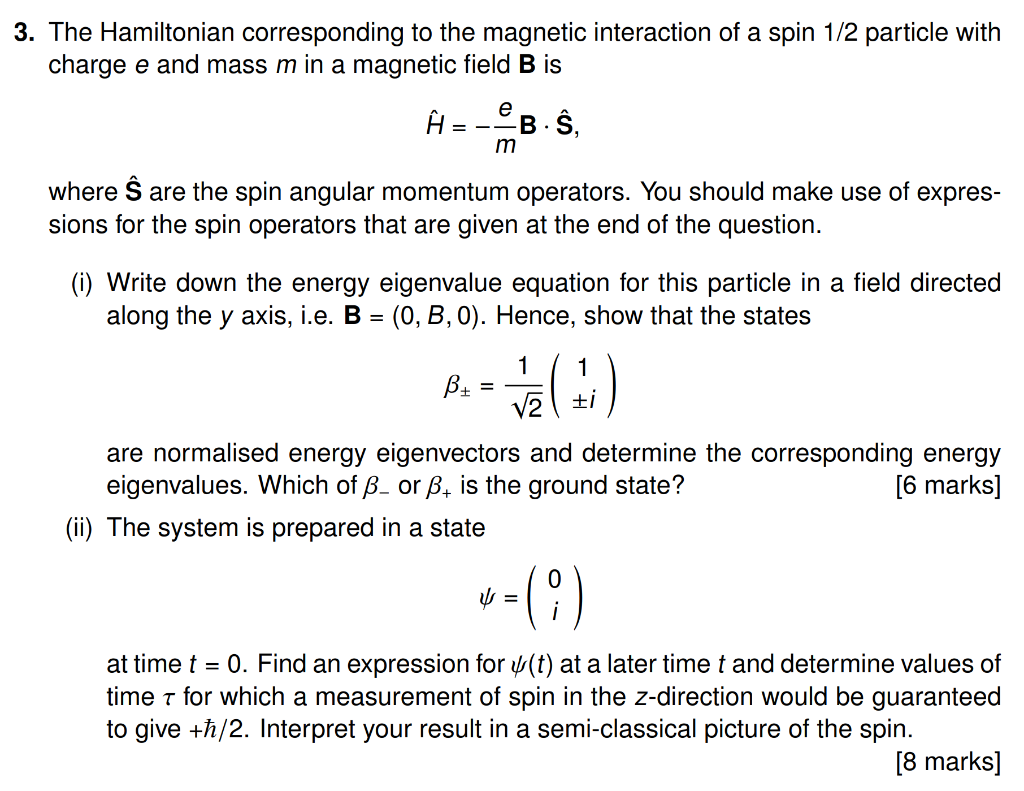qm 2019.3 3. The Hamiltonian corresponding to the magnetic interaction of a spin 1/2 particle with charge e and mass m in a magnetic field B is À eB B. Ŝ, m where Ŝ are the spin angular momentum operators. You should make use of expres- sions for the spin operators that are given at the end of the question. (i) Write down the energy eigenvalue equation for this particle in a field directed along the y axis, i.e. B...

• ### Could someone help me with question 2a) and also help with b), I can do 2b)...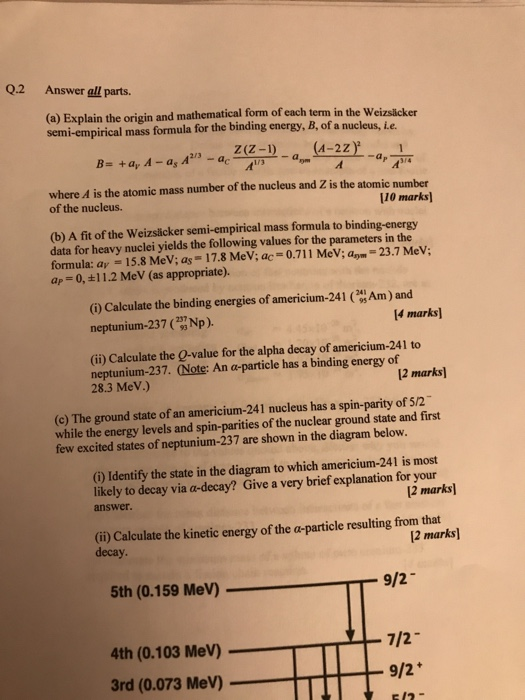Could someone help me with question 2a) and also help with b), I can do 2b) but I am wondering when do I use Ap = 0 or +/-11.2. Many thanks in advance. Q.2 Answer all parts. (a) Explain the origin and mathematical form of each term in the Weizslicker semi-empirical mass formula for the binding energy, B, of a nucleus, Le. Z(Z-1) (A-2Z B- +a, A - a A where A is the atomic mass number of the nucleus...

• ### I don't know if this one question counts as multiple questions. If it does, please help...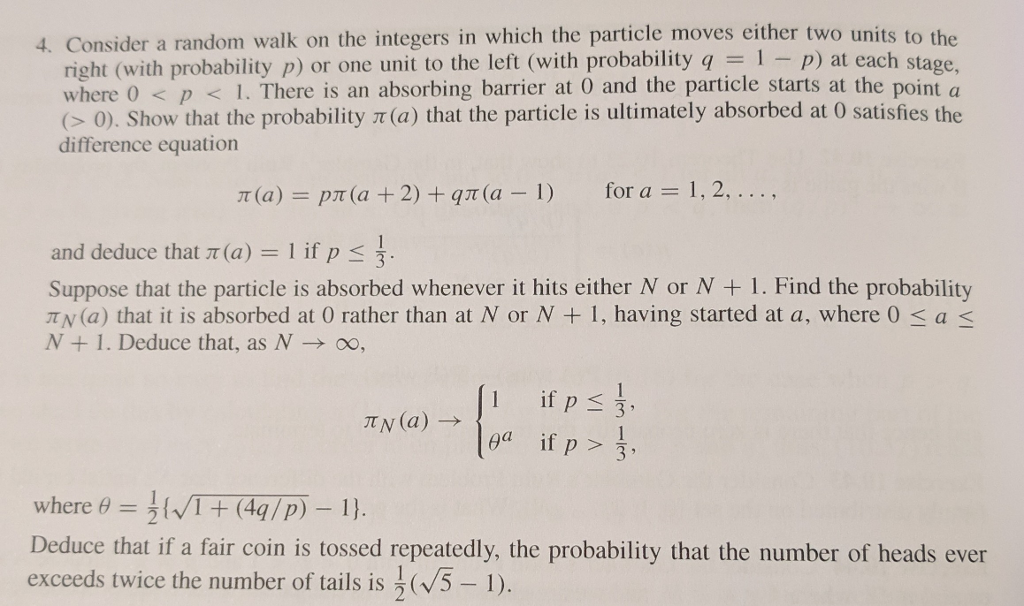I don't know if this one question counts as multiple questions. If it does, please help me with the first part and tell me that it counts as multiple parts. 4. Consider a random walk on the integers in which the particle moves either two units to the right (with probability p) or one unit to the left (with probability q1 p) at each stage where O < p1. There is an absorbing barrier at 0 and the particle starts...